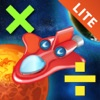# Space Mathematics: Multiplication and Division â€” Lite - By Rucarta - Lumos Educational App Store3.51
Price -Free
\$0

#### DESCRIPTION:

Space Mathematics: Multiplication and Division is a fun and effective way for kids to learn basic mathematical operations of multiplication and division! Any child is nearly guaranteed to learn multiplication and division table through the daily practice using this app at least 2 hours a day. No more need to remember an infinite number of tricks to multiply or divide two numbers. Just practice with this app and you'll remember them forever!!! Developed by professional school teachers Space Mathematics: Multiplication and Division is a fun game and educational app in one. The aim of the gam

#### OVERVIEW:

Space Mathematics: Multiplication and Division â€” Lite - By Rucarta is a free educational mobile app By Rucarta.It helps students in grades 3 practice the following standards 3.OA.A.4.

This page not only allows students and teachers download Space Mathematics: Multiplication and Division â€” Lite - By Rucarta but also find engaging Sample Questions, Videos, Pins, Worksheets, Books related to the following topics.

1. 3.OA.A.4 : Determine the unknown whole number in a multiplication or division equation relating three whole numbers. For example, determine the unknown number that makes the equation true in each of the equations 8 × ? = 48, 5 = __÷ 3, 6 × 6 = ?..

3

3.OA.A.4

#### ADDITIONAL INFORMATION:

Developer: Rucarta

Software Version: 1.3

Category: Education

### RELATED APPSEdSearch WebSearch